# How to approach a power-supply design – part 5

In this final installment of the topology blog series, I’ll introduce the inverting buck-boost converter and Ćuk converter. Both topologies allow you to generate a negative output voltage from a positive input voltage.

Inverting buck-boost converters

The inverting buck-boost topology can step up and step down its input voltage while the output voltage is negative. The energy transfers from the input to the output when switch Q1 is not conducting. Figure 1 shows the schematic of a nonsynchronous inverting buck-boost converter.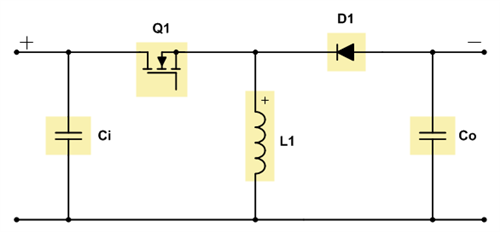Figure 1: Schematic of a nonsynchronous inverting buck-boost converter

Equation 1 calculates the duty cycle in continuous conduction mode (CCM) as: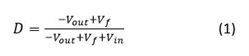Equation 2 calculates the maximum metal-oxide semiconductor field-effect transistor (MOSFET) stress as: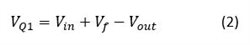Equation 3 gives the maximum diode stress as: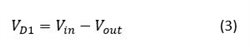where Vin is the input voltage, Vout is the output voltage and Vf is the diode forward. The value for Vout needs to be negative for all three equations.

Since there is no inductor-capacitor (LC) filter pointing to the input or output of the inverting buck-boost converter, this topology has pulsed currents at both converter ends, leading to rather high voltage ripple. For electromagnetic interference (EMI) compliance, additional input filtering might be necessary. If the converter needs to supply a very sensitive load, a second-stage filter at the output might not provide enough damping of the output voltage ripple. In such cases I recommend using a Ćuk converter instead.

You can build an inverting buck-boost converter by using a buck controller or converter as you need a P-channel MOSFET or high-side MOSFET driver. However, the ground terminals of the controller or converter integrated circuit (IC) need to be connected to the negative output voltage. The IC is then regulating the ground signal versus the negative output voltage.

The right half-plane zero (RHPZ) is the limiting factor for the inverting buck-boost converter’s achievable regulation bandwidth. The maximum bandwidth is roughly one-fifth the RHPZ frequency. Equation 4 estimates the single RHPZ frequency of the inverting buck-boost converter’s transfer function: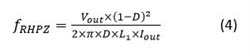where Vout is the output voltage, D is the duty cycle, Iout is the output current and L1 is the inductance of inductor L1. The values for both Vout and Iout need to be negative.

Figures 2 through 7 show voltage and current waveforms in CCM for the FET Q1, inductor L1 and diode D1 in a nonsynchronous inverting buck-boost converter.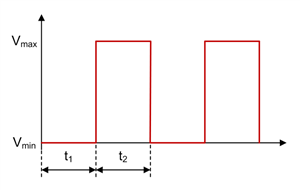Figure 2: Inverting buck-boost FET Q1 voltage waveform in CCM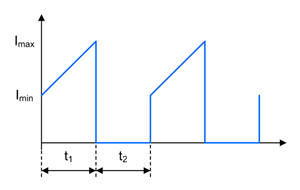Figure 3: Inverting buck-boost FET Q1 current waveform in CCM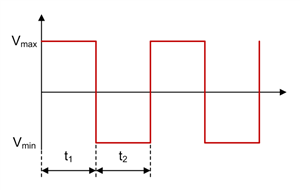Figure 4: Inverting buck-boost inductor L1 voltage waveform in CCM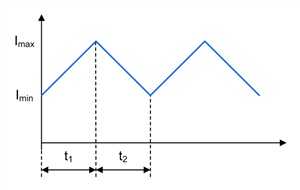Figure 5: Inverting buck-boost inductor L1 current waveform in CCM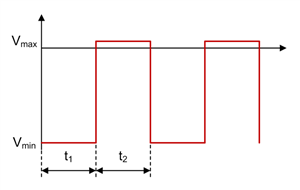Figure 6: Inverting buck-boost diode D1 voltage waveform in CCM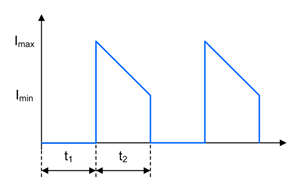Figure 7: Inverting buck-boost diode D1 current waveform in CCM

Ćuk converters

The Ćuk topology can step up and step down its input voltage while the output voltage is negative. The energy transfers from the input to the output when switch Q1 is not conducting. Figure 8 shows the schematic of a nonsynchronous Ćuk converter.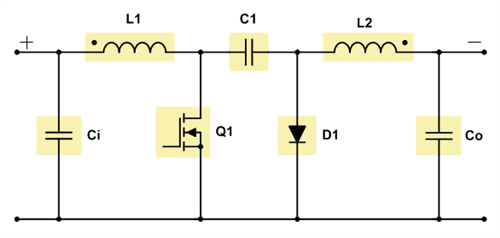Figure 8: Schematic of a nonsynchronous Cuk converter

Equation 5 calculates the duty cycle in CCM as: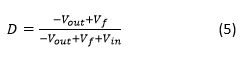Equation 6 calculates the maximum MOSFET stress as: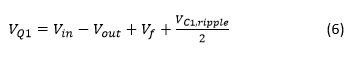Equation 7 gives the maximum diode stress as: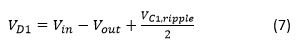where Vin is the input voltage, Vout is the output voltage, Vf is the diode forward voltage and VC1,ripple is the voltage ripple across the coupling capacitor C1. The value for Vout needs to be negative for these three equations.

The LC filter L2/Co in a Ćuk converter is pointing to the output. As a result, the output ripple is quite small, because the output current is continuous. When you look at the input, there is another LC filter with L1/Ci. Thus the input current is continuous as well, which results in very small input ripple, too. Thus the Ćuk converter is a perfect fit for applications that require a negative output voltage while being very sensitive at both the input and output, such as power supplies for telecommunications.

You can easily build a Ćuk converter by using a boost controller, because MOSFET Q1 needs to be driven on the low side. The boost converter or controller IC will typically only accept a positive feedback voltage at its feedback pin. You can translate the negative output voltage into a positive voltage signal by using a simple inverting operational amplifier circuit.

The Ćuk converter has an RHPZ as well. The power stage cannot immediately react to changes at the output, because the energy transfers to the output when switch Q1 is off. The maximum achievable crossover frequency is again one-fifth the RHPZ frequency. Note that a Ćuk converter has more than one RHPZ. Equation 8 estimates one of the Ćuk converter’s RHPZs as:where D is the duty cycle, L1 is the inductance of inductor L1 and C1 is the capacitance of coupling capacitor C1.

Figures 9 through 18 show voltage and current waveforms in CCM for the FET Q1, inductor L1, coupling capacitor C1, diode D1 and inductor L2 in a nonsynchronous Ćuk converter.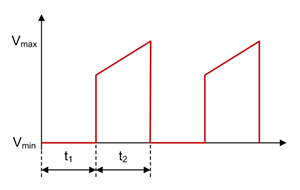Figure 9: Ćuk FET Q1 voltage waveform in CCM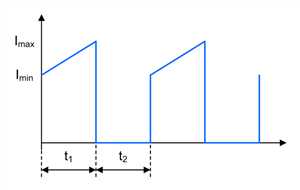Figure 10: Ćuk FET Q1 current waveform in CCM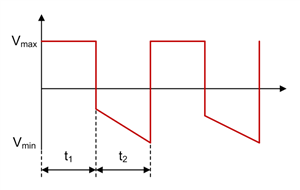Figure 11: Ćuk inductor L1 voltage waveform in CCM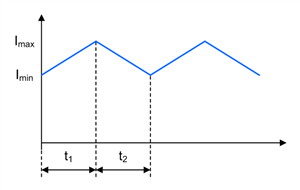Figure 12: Ćuk inductor L1 current waveform in CCM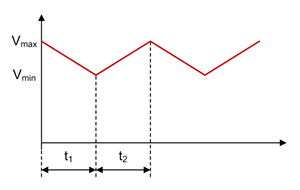Figure 13: Ćuk coupling capacitor C1 voltage waveform in CCM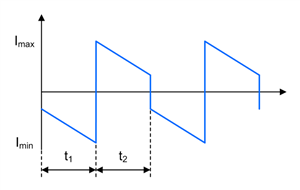Figure 14: Ćuk coupling capacitor C1 current waveform in CCM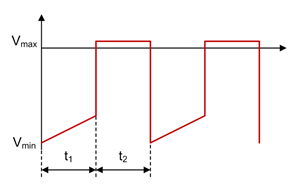Figure 15: Ćuk diode D1 voltage waveform in CCM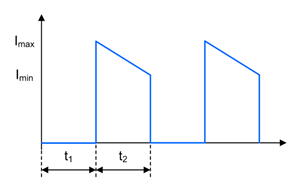Figure 16: Ćuk diode D1 current waveform in CCM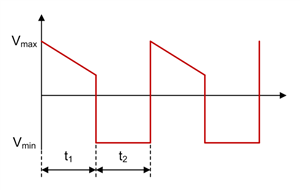Figure 17: Ćuk inductor L2 voltage waveform in CCM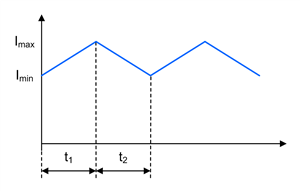Figure 18: Ćuk inductor L2 current waveform in CCM

Much like for a single-ended primary inductance converter (SEPIC) and Zeta converter, it can also make sense to use coupled inductors for a Ćuk converter instead of two separate inductors. Using coupled inductors offers two advantages: The first benefit is that only half the inductance compared to a two-inductor solution will be required for similar current ripple because coupling the windings leads to ripple cancellation. The second benefit is that you can get rid of resonance in the transfer function caused by the two inductors and the coupling capacitor. This resonance usually needs to be damped by a resistor-capacitor (RC) network in parallel with coupling capacitor C1.

One drawback to using coupled inductors is that you need to use the same inductance value for both inductors. Another limitation of coupled inductors is typically their current rating. For applications with high output currents, you might need to use single inductors.

You can also configure both inverting buck-boost and Ćuk converters as a converter with synchronous rectification if your application requires output currents greater than 3A. If you implement synchronous rectification for a Ćuk converter, you need to AC-couple the high-side gate-drive signal, because many controllers require you to connect them to the switch node. The Ćuk converter has two switch nodes, so take care to avoid negative voltage rating violations on the SW pin.